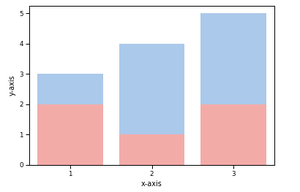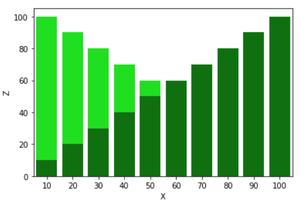How to overlap two Barplots in Seaborn?

• Last Updated : 25 Jan, 2022

Seaborn is an amazing visualization library for statistical graphics plotting in Python. It provides beautiful default styles and colour palettes to make statistical plots more attractive. It is built on the top of matplotlib library and also closely integrated to the data structures from pandas.

Bar Plot is used to represent categories of data using rectangular bars. We can overlap two barplots in seaborn by creating subplots.

Steps required to overlap two barplots in seaborn:

1. Importing seaborn and matplotlib library,  seaborn for plotting graph and matplotlib for using subplot().
2. Creating dataframe.
3. Creating two subplots on the same axes.
4. Displaying the plot.

Below are some examples based on the above approach:

Example 1:

Python3

 # importing all required librariesimport pandas as pdimport seaborn as snsimport matplotlib.pyplot as plt # creating dataframedf = pd.DataFrame({    'X': [1, 2, 3],    'Y': [3, 4, 5],    'Z': [2, 1, 2]}) # creating subplotsax = plt.subplots() # plotting columnsax = sns.barplot(x=df["X"], y=df["Y"], color='b')ax = sns.barplot(x=df["X"], y=df["Z"], color='r') # renaming the axesax.set(xlabel="x-axis", ylabel="y-axis") # visualizing illustrationplt.show()

Output:Example 2:

Python3

 #importing all required librariesimport pandas as pdimport seaborn as snsimport matplotlib.pyplot as plt #creating dataframedf=pd.DataFrame({    'X':[i for i in range(10,110,10)],    'Y':[i for i in range(100,0,-10)],    'Z':[i for i in range(10,110,10)]}) #creating subplotsax=plt.subplots() #plotting columnsax=sns.barplot(x=df["X"],y=df["Y"],color = 'lime')ax=sns.barplot(x=df["X"],y=df["Z"],color = 'green') #renaming the axesax.set(xlabel="x-axis", ylabel="y-axis") # visualizing illustrationplt.show()

Output:My Personal Notes arrow_drop_up